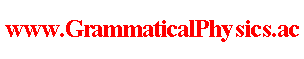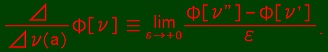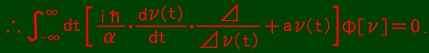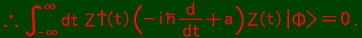since 2006 Help　Sitemap < Forum > < Products > < Quantum History Theory > < Uda Equation of Fermionic Mechanics > The content of this page is toward Uda equation of Free Dirac Field and was developed in Uda Equation of Dirac Field @ Quantum History Theory @ Problems. In this page, I treat a system of two real degrees x and y of freedom. I let the Hamiltonian H of this system be as H = a(x2 + y2). At first, I define a complex variable z as z = x + iy. Then H = az*z where z* is the complex conjugate of z. Quantization of this system is done as follows. X is an Hermitian operator whose eigenvalue is x. Y is an Hermitian operator whose eigenvalue is y. Z ≡ X + iY. [Z, Z]+ ≡ ZZ + ZZ = 0 ∴ Z2 = 0. [Z, Z†]+ ≡ ZZ† + Z†Z = 1. H = aZ†Z. Z2 = 0 ⇔ X2 - Y2 + iXY + iYX = 0 ⇔ [X, Y]+ = i(X2 - Y2). ZZ† + Z†Z = 1 ⇔ 2X2 + 2Y2 = 1 ⇔ X2 + Y2 = 1/2. H = aZ†Z = a(X2 + Y2 + iXY - iYX) = a(i[X, Y]- + 1/2). Z†Z|n> = n|n>, = 1, n ∈ R. n2 = n2(|n>, |n>) = (n|n>, n|n>) = (Z†Z|n>, Z†Z|n>) = = = 　∵ Z2 = 0 ∴ n2 = n = n ∴ n = 0 or 1. (Z|0>, Z|0>) = <0|Z†Z|0> = 0<0|0> = 0 ∵ Z†Z|0> = 0|0>. ∴ Z|0> = 0. (Z†|1>, Z†|1>) = <1|ZZ†|1> = <1|(1 - Z†Z)|1> = 0 ∵ Z†Z|1> = |1>. ∴ Z†|1> = 0. Z†ZZ†|0> = Z†(1 - Z†Z)|0> = Z†|0> ∵ Z|0> = 0. (Z†|0>, Z†|0>) = <0|ZZ†|0> = <0|(1 - Z†Z)|0> = <0|0> = 1 ∵ Z|0> = 0. ∴ Z†|0> = |1>. Z†ZZ|1> = 0 = 0Z|1> ∵ Z2 = 0. (Z|1>, Z|1>) = <1|Z†Z|1> = <1|1> = 1 ∵ Z†Z|1> = |1>. ∴ Z|1> = |0>. As a summary, Z|0> = 0, Z|1> = |0>, Z†|0> = |1>, Z†|1> = 0. The above explanations are about quantum state. Now I start explaining my theory about quantum history. A function which represents a classical history. ν: R → {0,1}. ν(t) = 0 or 1. A basis of the quantum history vector space: |ν>. A quantum history vector: ∫Dν Φ[ν] |ν>. An operator which acts on a specific time: Z†(t)Z(t)|ν> = ν(t)|ν>. Uda Equation: 　ih limε→+0 (1/ε)∫Dν{Φ[ν(□ - ε)] - Φ[ν]}|ν> = αa∫-∞∞dt Z†(t)Z(t)∫Dν Φ[ν] |ν> 　∴ ih limε→+0 (1/ε){Φ[ν(□ - ε)] - Φ[ν]} = αa∫-∞∞dt ν(t)Φ[ν].ν'(t) = ν''(t) = ν(t) if t ＜ a or t ＞ a + ε. ν'(t) = 0 and ν''(t) = 1 if a ≦ t ≦ a + ε. Uda equation: 　-ih∫-∞∞dt [dν(t)/dt][⊿/⊿ν(t)]Φ[ν] = αa∫-∞∞dt ν(t)Φ[ν].Another formulation can stand as follows. At first, I put a condition [Z(t), Z†(t')]+ = δ(t - t'), [Z(t), Z(t')]+ = 0. This condition realizes that [Z†(t)Z(t), Z†(t')Z(t')]- = 0. Then I replace limε→+0 (1/ε)∫Dν {Φ[ν(□ - ε)] - Φ[ν]} |ν> with ∫-∞∞dt Z†(t)(d/dt)Z(t)∫Dν Φ[ν] |ν>. Uda equation: 　ih∫-∞∞dt Z†(t)(d/dt)Z(t) |Φ> = a∫-∞∞dt Z†(t)Z(t) |Φ>I removed factor α heuristically by seeing dimensionality. Below I explain what ∫-∞∞dt Z†(t)(d/dt)Z(t) |Φ> means. ∫-∞∞dt Z†(t)(d/dt)Z(t) |Φ> = limε→0 (1/ε)∫-∞∞dt Z†(t)[Z(t + ε) - Z(t)] |Φ> = limε→0 (1/ε)∫-∞∞dt [Z†(t - ε) - Z†(t)]Z(t) |Φ> Z†(t - ε)Z(t) creates a particle at time t - ε after annihilating a particle at time t only if there is a particle at time t. Z†(t)Z(t) creates a particle at time t after annihilating a particle at time t only if there is a particle at time t. So, limε→0 (1/ε) [Z†(t - ε) - Z†(t)]Z(t) |Φ> is a kind of partial differentiation only if there is a particle at time t. limε→0 (1/ε) [Z†(t - ε) - Z†(t)]Z(t) |Φ> = 0 if there is no particle at time t. As for partial differentiation, the following formula holds. limε→0 (1/ε) [F(x1 +ε, ・・・, xn + ε) - F(x1, ・・・, xn)] = Σk=1n limε→0 (1/ε) [F(x1, ・・・, xk-1, xk + ε, xk+1, ・・・, xn) - F(x1, ・・・, xn)] On the analogy of this, the variation of |Φ> caused by shifting all times when there is a particle by -ε is thought to be ε∫-∞∞dt Z†(t)(d/dt)Z(t) |Φ> as ε → 0. If a quantum history is not entangled in time-like direction at all, to shift all times when there is a particle by -ε is to replace quantum state at time t with quantum state at time t + ε for all t. This is the very concept of left hand side of Uda equation. Author Yuichi Uda, Write start at 2019/05/17/16:03JST, Last edit at 2019/05/20/16:27JST